• 单链表的创建插入删除
2022-07-19 23:32:23

单链表：线性表的链式存储，通过任意的存储单元来存储线性表中的数据元素。

 data 数据 next 指针

优点：不需要大片的连续的空间，改变容量方便。

缺点：不可随机读取，要耗费一定空间存放指针。

# 一、单链表的创建

1、头插法

LinkList List_HeadInsert(LinkList &L){
LNode *s;
int x;
L->next=NULL;
scanf("%d",&x);
while(x!=999){
s=(LNode*)malloc(sizeof(LNode));
s->data=x;
s->next=L->next;
L->next=s;
scanf("%d",&x);
//TODO
}
return L;
}

2、尾插法

LinkList List_TailInsert(LinkList &L){
int x;
LNode *s,*r;
s,r=L;
scanf("%d",&x);
while(x!=999){
s=(LNode*)malloc(sizeof(LNode));
s->data = x;
r->next = s;
r = s;
scanf("%d",&x);
//TODO
}
r->next = NULL;//尾结点指针置空
return L;
} 

# 二、单链表的查找

1、按位查找

LNode *GetElem(LinkList &L,int i){
int j=1;
LNode *p=L->next;
if(i==0){
return L;
//TODO

}
if(i<0){
return NULL;
//TODO
}
while(p!=NULL&&j<i){
p=p->next;
j++;
//TODO
}
//	printf("%d\n",p->data);
return p;
}

2、按值查找

LNode *LoacateElem(LinkList &L,int e){
LNode *p=L->next;
while(p!=NULL&&p->data!=e){
p=p->next;
//TODO
}
return p;
} 

## 三、单链表的插入

1、按位插入

bool Insert(LinkList &L,int i,int e){
LNode *p = GetElem(L,i-1);
LNode *s = (LNode*)malloc(sizeof(LNode));//开辟成功就返回该空间的首地址 因为malloc函数的返回值为void*，所以需要强制类型转换为对应类型。
s->data = e;
s->next = p->next;
p->next = s;
return true;
}

2、按节点插入

bool ForeInsert(LNode *p,int e){//若使用给定节点的前插操作，事件复杂度O(n)
if(p==NULL){		//这里采用先将要插的节点插入p的后面，然后再交换数据的方法，事件复杂度为O(1)
return false;
//TODO
}
LNode *s = (LNode*)malloc(sizeof(LNode));
if(s==NULL){
return false;
//TODO
}
s->next = p->next;
p->next = s;
s->data = p->data;
p->data = e;
return true;
}

# 四、单链表的删除

1、按位删除

bool DeleteList(LinkList &L,int i,int &e){
LNode *p = GetElem(L,i-1);
LNode *q = GetElem(L,i);
p->next = q->next;
e = q->data;
free(q);
return true;
}

2、按节点删除

bool DeleteNode(LNode *p){//指向最后一个节点时需要特殊处理
if(p==NULL){
return false;
//TODO
}
LNode *q = p->next;
p->data = q->data;
p->next = q->next;
free(q);
return true;
}

五、单链表的长度

int length(LinkList &L){
int length = 0;
LNode *p = L;
while(p->next!=NULL){
p=p->next;
length++;
//TODO
}
return length;
}数据结构
更多相关内容
• 单链表 创建 插入 删除 长度 销毁 ，自己写的！看看能帮到你不
• 单链表创建插入删除 1、 以正序或逆序方式动态创建一个单链表，输入数据并验证是否成功； 2、 在原有单链表的基础上，实现在指定位置上对某个数据元素的插入，其中插入位置不限（可以是表头、表尾，也可以是...

## 标题单链表的创建，插入，删除

1、 以正序或逆序方式动态创建一个单链表，输入数据并验证是否成功；
2、 在原有单链表的基础上，实现在指定位置上对某个数据元素的插入，其中插入位置不限（可以是表头、表尾，也可以是其中的任何一个位置），但要求在有效范围内，输入数据并验证是否成功；3、 在原有单链表的基础上，实现删除指定位置上的某个数据元素，返回删除的数据元素值，其中删除位置不限，但要求在有效范围内，输入数据并验证是否删除成功；4、 查找单链表中第i个元素的值。5、 输出单链表中所有元素的值。

代码如下：

#include<stdlib.h>
#include<stdio.h>
#include
using namespace std;
//定义单链表节点数据类型
typedef struct LNode
{
int data;
struct LNode* next;
}LNode,List;
//以逆序方式动态创建一个单链表，输入数据并验证是否成功；
//创建一个单链表（带头结点）
List CreateList(List& L,int n)
{
for(int i=0;i<n;i++)
{
LNode
p = (LNode*)malloc(sizeof(LNode));//新建结点
scanf_s ("%d",&p->data);
p->next = L->next ;
L->next = p;
}
cout << “单链表创建成功！” << endl;
return L;
}
//输出单链表
void print(LNode* L,LNodep)
{
while(p != NULL)
{
cout << p->data<<" ";
p= p->next;
}
cout << endl;
}
//在第i个位置前插入int类型元素e
void ListInsert(LNode
L, int i, int e)
{
if (i < 1)
{
cout << “插入不合法” << endl;
system(“pause”);
}
LNode* p;
int j = 0;
p = L;
while (p != NULL && j < i - 1)
{
p = p->next; j++;
}
if (p == NULL) { system(“pause”); }
LNode* s = (LNode*)malloc(sizeof(int));
s->data = e;
s->next = p->next;
p->next = s;
return ;
}

//删除位序为i的数据
int ListDelete(LNode* L, int i)
{
int e;
LNode* p;
int j = 0;
p = L;
while (p!=NULL&&j<i-1)
{
p = p->next;
j++;
}
if (p == NULL)
system(“pause”);
if (p->next == NULL)
system(“pause”);
LNode* q = p->next;
e = q->data;
p->next = q-> next;
free(q);
return e;
}
//查找第i个元素 并返回
int GetElem(List L, int i)
{
int j = 1;
LNode* p = L->next;
if (i == 0)
system(“pause”);
if (i < 1)
return NULL;
while (p != NULL && j < i)
{
p = p->next;
j++;
}
return p->data;
}
int main()
{
List L;
L = (LNode*)malloc(sizeof(LNode));//给头结点分配内存空间
L->next = NULL;//建立一个带头结点的空链表
cout << “请输入数据” << endl;
L = CreateList(L, 5);
LNode* p = L->next;
print(L, p);
cout << “单链表输出完成！” << endl;
//插入数据
cout << “开始在第i个位置插入元素e” << endl;
int i, e;
cout << “请给i赋值”; cin >> i;
cout << “请给e赋值”; cin >> e;
ListInsert(L, i, e);

运行结果：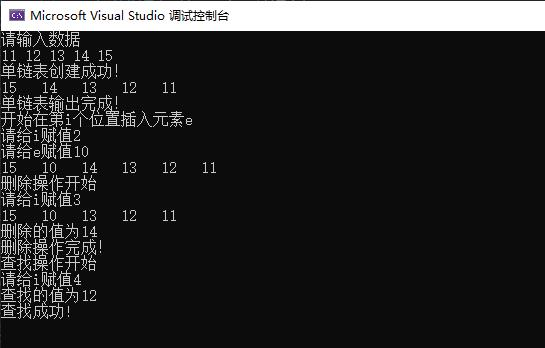展开全文数据结构
• 要实现对单链表中节点的插入删除与查找的功能，就要先进行的单链表的初始化、创建和遍历，进而实现各功能，以下是对单链表节点的插入删除、查找功能的具体实现： #include #include #include typedef int ...
• 单链表 单链表是一种链式存取的数据结构，用一组地址任意的存储单元存放线性表中的数据元素。...单链表创建和输出 #include<stdio.h> #include<stdlib.h> #define LEN sizeof(struct Stud

## 单链表

单链表是一种链式存取的数据结构，用一组地址任意的存储单元存放线性表中的数据元素。链表中的数据是以结点来表示的，每个结点的构成：元素(数据元素的映象) + 指针(指示后继元素存储位置)，元素就是存储数据的存储单元，指针就是连接每个结点的地址数据。

（注意代码中的注释很重要！！！）以下代码均默认用户正确输入。

## 单链表的创建和输出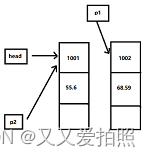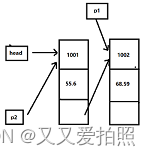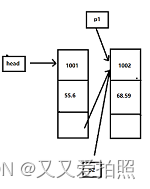#include<stdio.h>
#include<stdlib.h>
#define LEN sizeof(struct Student)

struct Student{
long num;
float score;
struct Student* next;
};        //分号!! 特别容易忘!!
int n;//n为节点数
//建立链表函数,creat函数带回一个起始地址
struct Student* creat(void){
struct Student* p1,* p2;
n=0;
//开辟新单元
// 编译系统会实现隐式类型转换，所以也可以写成p1=malloc(LEN);
p1=p2=(struct Student*)malloc(LEN);
//输入第一个学生的学号和成绩
scanf("%ld,%f",&p1->num,&p1->score);
while (p1->num){
n+=1;
else p2->next=p1;
p2=p1;
p1=(struct Student*)malloc(LEN);
//输入其他学生的学号和成绩
scanf("%ld,%f",&p1->num,&p1->score);
}
p2->next=NULL;
}

// 输出链表的函数
void print(struct Student* head){
struct Student* p;
printf("Now,There %d record are :\n",n);
do{
printf("%ld %.1f\n",p->num,p->score);
p=p->next;
}while(p!=NULL);
}
}

int main(){
return 0;
}


输入：

1001,55.6
1002,68.59
1003,88.6
0

输出：

Now,There 3 record are :
1001 55.6
1002 68.6
1003 88.6

## 单链表的插入：

头插法：（在上面创建的基础上，只贴了部分代码）
在头部插入结点，将头指针指向新结点。在将新结点指向原来的第一个结点。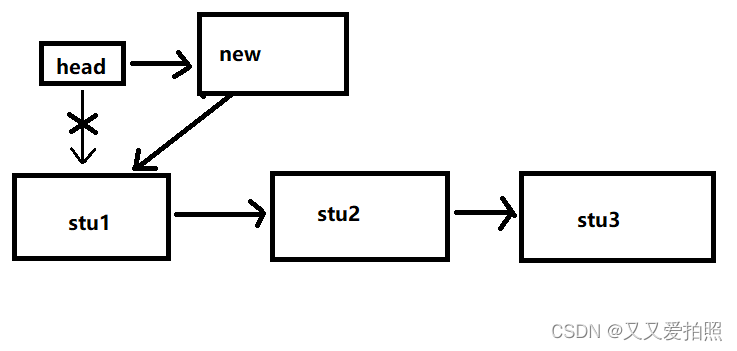#include<stdio.h>
#include<stdlib.h>
#define LEN sizeof(struct Student)

int n
//需要插入的学生
void getInput(struct Student *stu){
printf("请输入学生及分数");
scanf("%ld,%f",&stu->num,&stu->score);
}
//执行插入操作
struct Student* stu,*temp;
stu=(struct Student*)malloc(sizeof(struct Student));
if(stu==NULL){
printf("内存分配失败");
exit(1);
}
getInput(stu);
{
stu->next=temp;
}else{
//如果链表里面没有数据
stu->next=NULL;
}
n++;
}
int main(){
return 0;
}


中间插入：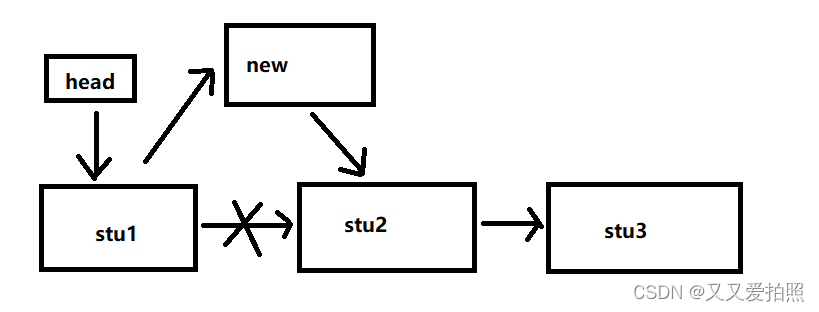//需要插入的学生
void getInput(struct Student *stu){
printf("请输入学生及分数:");
scanf("%ld,%f",&stu->num,&stu->score);
}
//执行插入操作
//需要插入位置的前一个结点
struct Student *previous;
//需要插入的结点
struct Student *current;
//需要插入位置的后一个结点
struct Student *new;
previous=NULL;
int count=1;
while (current!=NULL&&count<=num)
{
count++;
previous=current;
current=current->next;
}
new=(struct Student*)malloc(sizeof(struct Student));
if(new==NULL){
printf("内存分配失败");
exit(1);
}
getInput(new);
//如果链表里面没有数据
if(previous==NULL){
}else{
previous->next=new;
}
n++;
new->next=current;
}


尾插法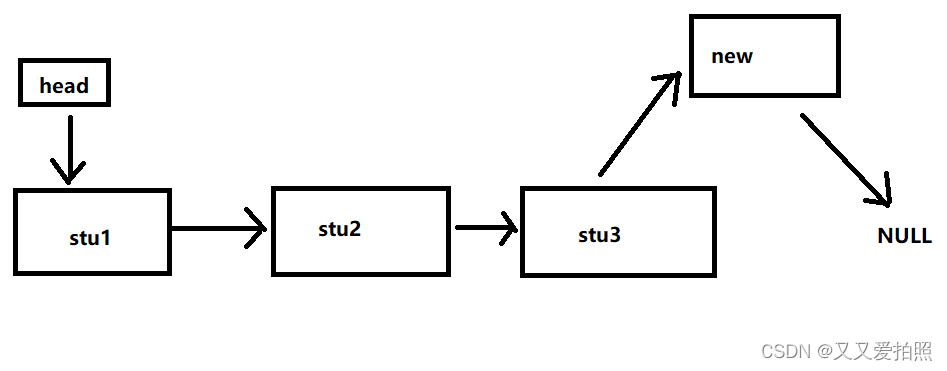//在尾部插入指针
struct Student* stu,*temp;
stu=(struct Student*)malloc(sizeof(struct Student));
if(stu==NULL){
printf("内存分配失败");
exit(1);
}
getInput(stu);
{
// 定位单链表的尾部位置
while (temp->next !=NULL)
{
temp=temp->next;
}
// 插入数据
temp->next=stu;
stu->next=NULL;
}else{
stu->next=NULL;
}
n++;
}


## 删除结点：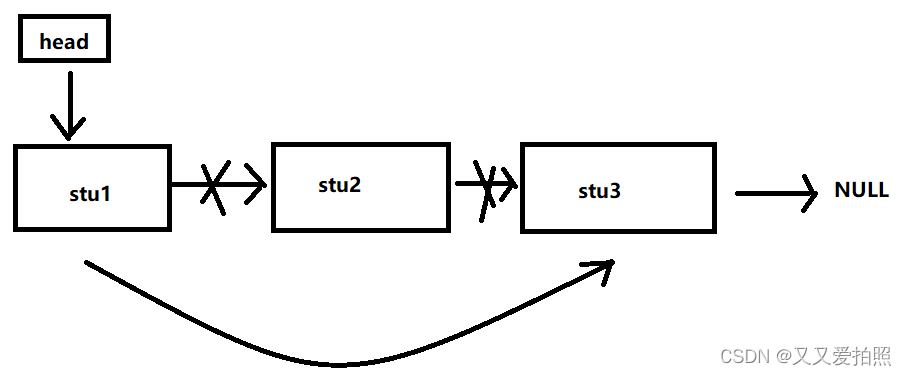// 删除结点
void delStu(struct Student **head,int num){
//需要删除位置的前一个结点
struct Student *previous;
//需要删除位置的后一个结点
struct Student *current;

previous=NULL;
int count=1;
while (current!=NULL&&count!=num)
{
count++;
previous=current;
current=current->next;
}
if(current==NULL){
printf("找不到匹配的结点");
return;
}else{
//需要删除的结点在第一个位置时
if(previous==NULL){
}else{
previous->next=current->next;
n--;
}
free(current);
}
}


展开全文数据结构
• 那么在这篇文章中，我们将要来讲解单链表插入删除操作。我们在上篇文章中已经讲解过，如果想要在表L中的第i个位置上插入指定元素e，我们需要找到第i-1的结点，在其后插入新的结点。大致步骤如下：当然当我们想在...

# 前言

在上一篇文章（单链表的定义）中我们已经了解了单链表的含义和简单的实现。那么在这篇文章中，我们将要来讲解单链表的插入和删除操作。

# 按位序插入（带头结点）

我们在上篇文章中已经讲解过，如果想要在表L中的第i个位置上插入指定元素e，我们需要找到第i-1的结点，在其后插入新的结点。大致步骤如下：

1. 使用malloc()函数申请一个新的结点
2. 往新的结点存入新的元素e
3. 将i-1位的元素指针指向新结点
4. 将新结点的指针指向原先第i个元素

当然当我们想在第一位插入新结点时，对于带头结点的链表的好处就是，在头部插入数据和其他任意一个位置插入数据的操作是一样的，非常方便。

## 代码实现

typedef struct LNode{
ElemType data;
struct LNode *next;

bool ListInsert(LinkList &L,int i,ElemType e){
if(i<i)
return false;
LNode *p;			// 指针p指向当前扫描到的结点
int j = 0;			// 当前p指针指向的是第几个结点
p = L;				// L指向头结点，头结点时第0个结点（不存放数据）
while(p!=NULL && j<i-1){
p = p->next;
j++;
}
if(p=NULL)			// i值不合法
return false;
LNode *s = (LNode *)malloc(sizeof(LNode));
s->data = e;
s->next = p->next;
p->next = s;		// 将结点s连接到p之后

return true;		// 插入成功
}


### 代码分析（在第一个位置插入结点）

若 i = 1

我们直接看ListInsert()函数的内部

1. 在进入时首先会判断，i的合法性（i小于1是不合法的）

• 位序是从1开始，不可能会比开头的还要小。如果小的话那就不属于链表中的元素。
2. 声明一个p指针，声明一个j变量用来表示p指针指向第几个结点

3. 紧接着将p指针指向头结点L

4. 这时候由于j = 0；i = 0不满足循环条件，所以直接不进行循环。

5. 判断i值是否合法

6. 创建一个新的结点，并且将结点的指针返回给*s

7. s->data：将e赋值给s的数据域（将e装入s）

8. s->next=p->next：将s的指针指向p指针指向的位置。

• 由于p指针在上面已经指向头结点了，所以p指针的next就跟头节点一样指向a1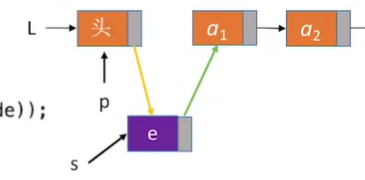9. p->next=s：将p指针的指针指向s

• 由于在第一个位置插入结点，所以链表中的第一个结点不再是a1，而是s了，所以需要将p的指针指向s。

注意：s->next=p->nextp->next=s的代码顺序不能颠倒。如果颠倒则会出现一下状况：

1. p先将指针指向s
2. s再将指针指向p指向的（也就是s）

就会形成一种“我指我自己”的尴尬局面。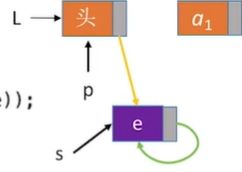### 代码分析（在第三个位置插入结点）

若 i = 3

我们直接从不同的地方说起：

1. 这时候则会进入while循环

• while循环的作用，其实就是将p指针指向下一个结点，直至指向想要插入的位置-1——i - 1为止。

• 未执行while循环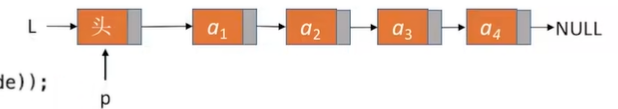• 执行1次while循环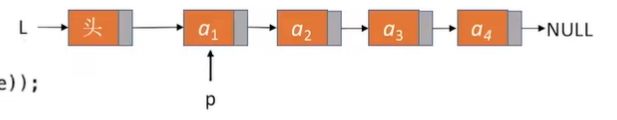• 以此类推，直至到想要的位置-1（这里是到第二个位置）

2. 紧接着下述的结点如何插入，与在第一个位置插入结点的操作相同，所以不多赘述

### 代码分析（在第六个位置插入结点）

由于该链表中只有4个结点（头结点不算），所以while循环最多只能循环5次——也就是当p指针指向a4结点的next——NULL时就会推出循环。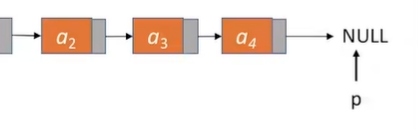并且在if判断的时候由于P==NULL所以会直接退出函数，并且返回false。

## 时间复杂度

最好情况：在第一个位置插入新结点，时间复杂度为O(1)

最坏情况：在最后一个位置+1插入新结点，时间复杂度为O(n)

平均情况：假设在每一个位置插入新结点是等可能事件，时间复杂度为O(n)

# 按位序插入（不带头结点）

其实不带头结点的插入原理和带头结点是一样的，都是先找到i-1位置上的结点，再进行插入。但是不带头结点的单链表在第一个位置插入元素时需要特殊处理。

## 代码实现

bool ListInsert(LinkList &L, int i, ELemType e){
if(i<1)
return false;
if(i==1){		// 在第一个位置插入结点
LNode *s = (LNode *)malloc(sizeof(LNode));
s->data = e;
s->next = L;
L = s;
return true;
}
LNode *p;		// 指针p指向当前扫描到的结点
int j = 1;		// 当前p指针指向的时第几个结点
p = L;			// p指针指向第一个结点（注意：不是头结点）
while(p!=NULL && j<i-1){	// 循环找到第i-1个结点
p = p->next;
j++;
}
if(p==NULL)		// 检测i合法性（是否超出链表长度）
return false;
LNode *s = (LNode *)malloc(sizeof(LNode));
s->data = e;
s->next = p->next;
p->next = s;

return true;	// 插入成功
}


### 代码分析（在第一个位置插入结点）

若 i = 1

1. 判断I的合法性，i是否是小于1（起始值）
2. 判断是否要在第一个位置插入节点
• 使用malloc()函数创建一个新的结点
• 将e的值存入新结点中
• 将新结点的指针指向原先链表中的第一个结点（头指针L在不带头结点的链表中代表的就是第一个结点）
• 由于在第一个位置插入了新的结点，所以应该将头指针L（指向第一个位置结点的指针）指向s
• 插入成功，返回true

从上述我们可以看出，如果是不带头结点的单链表的话，在第一个位置插入元素，需要改变头指针L指向新插入的结点。而在带头结点的单链表中，头指针始终都是指向头结点的，只需要改变头结点的指针指向新插入的元素，其实和在任意位置插入一个新的结点的操作是相同的，并不用专门写一段代码来对在第一个位置插入元素进行特殊处理。

从这里我们就可以看出带头结点的链表的代码便捷性。

### 代码分析（i>1）

不带头结点的链表，在除了第一个位置外的其他位置插入新结点，跟带头结点的链表是一样的，维度有一个不同就是在j的赋值上：

• 带头结点：是因为p指针刚开始指向的是头结点（头结点在链表中不算一个真正的结点，所以我们将其记为0），故将j赋值为0
• 不带头结点：由于p指针直接就是指向链表中的第一个结点，故直接将J赋值为1

# 指定结点的后插操作

后插的操作和按位序操作的区别就是：前者是给你一个结点的地址，你直接在该结点后插入一个结点，而后者是给你要插入结点的位序，你在该位序插入结点。

typedef struct LNode{
ElemType data;
struct LNode *next;

bool InsertNextNode(LNode *p,ElemType e){
if(P==NULL)
return false;
LNode *s = (LNode *)malloc(sizeof(LNode));
if(s==NULL)				// 内存分配失败
return false;
s->data = e;			// 将e存入新结点
s->next = p->next;
p->next = s;			// 将结点s连接到p之后

return true;
}


由于我们已经知道了i-1的地址，所以不需要再进行遍历去找到i-1的结点的位置。所以可以直接创建一个新的结点，进行存入数据和指针重指的操作（插入新指针的操作同上，不再赘述）

注意：if(s==NULL)是用来判断内存分配是否成功，若失败则退出并返回false

由于链表中插入结点的代码是一样的，那么再按位序插入的代码中，我们可以将插入结点的代码删去，直接改为调用InsertNextNode()函数

• 按位序插入和后插操作就是多了遍历和判断，后插操作只有插入结点的操作，所以我们可以在按位序插入的函数中直接调用后插操作，减少代码的冗余性

# 指定结点的前插操作

在单链表中我们知道，给定一个结点（p）后，我们可以知道这个结点后面的所有元素，但是无法知道前面的元素。如果给定一个地址，需要在该节点的前方插入结点的话，我们就需要头指针。通过头指针找到给定地址的结点（p）的前驱，在前驱后插入结点即可。

• 如何找到前驱：前驱的指针应该是指向给定地址的结点（p），当指针的地址和给定地址的结点的地址相等时，则代表当前结点为前驱

但是这样子的操作，时间复杂度为O(n)，那么还有没有另一种方法呢？

有的！

我们换一个思路，我们虽然不能将结点直接在前驱后插入，但是我们可以将数据对换。

1. 在给定地址的结点（p）后面插入一个结点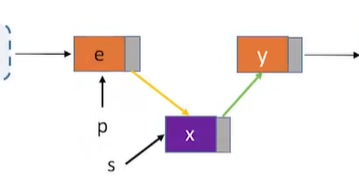2. 这时候重点来了，我们将结点p的数据值和新插入的结点的值对调

虽然我们无法找到p结点的前驱结点，但是在逻辑上，我们实现了前插操作，并且时间复杂度为O(1)

## 代码实现

bool InsertPriorNode(LNode *p,ElemType e){
if(p==NULL)
return false;
LNode *s = (LNode *)malloc(sizeof(LNode));
if(s==NULL)		// 内存分配失败
return false;
s->next = p->next;
p->next = s;		// 新结点s连接到p之后
s->data = p->data;	// 将p中元素赋值到s中
p->data = e;		// p中元素覆盖为e

return true;
}


因为我们进行的前插操作，也是要先将新结点插在p结点后，最后调换数据域，所以为了减少代码的冗余性，又由于我们知道插入新结点的操作已经可以由InsertNextNode()实现，那么我们调用该函数先创建一个新的结点，然后再将新的结点传入前插操作的函数。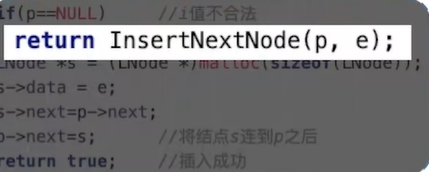bool InsertPriorNode(LNode *p,LNode *s){
if(p==NULL || s==NULL)
return false;
s->next = p->next;
p->next = s			// 将结点s连接到p之后
ElemType temp = p->data	// 交换数据域部分
p->data = s->data;
s->data = temp;

return true;
}


上述的新代码中*p是要在哪个结点前插入，*s是要插入的新结点。

# 按位序删除（带头结点）

## 代码实现

bool ListDelete(LinkList &L,int i,ElemType &e){
if(i<1)
return false;
LNode *p;
int j = 0;
p = L;
while(p!=NULL && j<i-1){
p = p->next;
j++;
}
if(p==NULL)
return false;
if(p->next==NULL)
return false;
LNode *q = p->next;
e = q->data;
p->next = q->next;
free(q);

return true;
}


由于，在if(p==NULL)的代码前面的部分，和按位序插入的操作一样，就是找到要删除的结点的前驱结点，所以在这里不再赘述。

重点我们看到LNode *q = p->next;及之后的代码。

1. 创建一个q指针，指向p的后继结点
• p指针在经过上述的操作后，已经指向要删除的结点的前驱结点，也就是将q指针指向要删除的结点
2. 使用e变量将要删除的值带回
• 注意：这里e是引用型变量
3. 将p的指针指向，q的后继
4. 使用free()释放q指针

## 时间复杂度

最坏、平均时间复杂度：O(n)

最好时间复杂度：O(1)

# 指定结点的删除

经过了上面前插操作，我们可以知道指定结点的删除也有两种方法：

p结点为要删除的结点，q指针为指向要删除的结点的指针

1. 方法一：传入头之间，遍历找到前驱结点
2. 方法二：将q指针，指向p结点的后继结点。将p结点的后继结点的数据域复制给p结点，并且将p结点的next指针指向p结点的后继节点的next，再使用free(q)释放结点

## 代码实现

bool DeleteNode(LNode *p){
if(p==NULL)
return false;
LNode *q = p->next;				// 令q指向*p的后继结点
p->data = p->next->data;		// 和后继结点交换数据域
p->next = q->next;				// 将*q结点从链中“断开”
free(q);						// 释放后继结点的存储空间
}


上述这种方法的时间复杂度为：O(1)

但是如果当我们要删除的结点是最后一个时，就会出现问题。如果真的遇到这种情况，那么就只能使用头指针的方式遍历找到前继结点再删除，所以上述的代码其实是有一些bug的，但是可以这么写大概会扣个1分。

# 单链表的局限性

从上述可以看出单链表的局限性：无法逆向检索，有时候不太方便。

# 结束语

本人菜鸟一枚，仅分享学习笔记和经验。若有错误欢迎指出！共同学习、共同进步 😃

如果您觉得我的文章对您有所帮助，希望可以点个赞和关注，支持一下！十分感谢~（若您不想也没关系，只要文章能够对您有所帮助就是我最大的动力！）

下一篇文章传送门：正在更新，敬请期待…

展开全文数据结构
• 单链表创建插入删除，存在小问题，提出来，多交流
• 本实验主要完成单链表基本操作的实现，包括单链表的初始化操作，前（后）插法建表，取值，单链表的查找，单链表插入删除操作 本实验约定单链表中存储的是整型数据 */ #include <bits/stdc++.h> using ...
• （1）通过传入头指针的方式实现 （2）通过复制插入位置的结点方式实现 二、删除操作 （一）按位序删除（带头结点） ListDelete(&L,i,&e)：删除操作。删除表L中第i个位置的元素，并用e返回删除元素的值。 找到第 i-1...数据结构
• 单链表基本操作 1.初始化 1）创建结构体，储存节点的数据+指针域； 2）创建指向结构体的指针； 3）用结构体定义单链表； 4）初始化链表头节点：为头节点开辟内存； 5）判断内存是否开辟成功； 6）头节点的指针域...数据结构 acm竞赛
• 本期推文主要给大家介绍数据结构中单链表插入删除操作的原理以及其具体的实现过程，学会单链表创建以及其具体操作实现 建立单链表 1） 头插法建表 将新结点逐个插入链表的头结点之后来创建链表，所以，得到的...
• 1.创建一个简单的单链表 #include <stdio.h> /* *csdn学院 *目的：让代码见证成长(作为一个初学的菜鸟，如 *大家有发现错误，欢迎指正！) *运行软件：CodeBlocks *作者:小臣小仁 *完成日期:2020年3月...
•数据结构
• [c]代码库//单链表插入删除#include#includetypedef struct node{char data;struct node *next;}LNode;//创建单链表LNode *create(){LNode *head,*p,*q;char x;head=(LNode*)malloc(sizeof(LNode));head->...
• 链表的插入排序，注意对于单链表插入删除，找到待插入或者待删除的结点的前驱是很重要的，注释的很清楚了，参考注释，有错误恳请指正！数据结构
• 1.在第i个元素之前插入元素数据e： while(p && j<i-1){p=p->next;++j} while循环中找到第i-1个结点，此时p代表第i-1个结点的地址。仅判断p不为null。不需要判断p->next，p->next是否为null与...数据结构
• 实现单链表的基本操作： #include<stdio.h> #include<malloc.h> //动态存储分配头文件 #define OK 1 #define ERROR 0 #define TRUE 1 #define FALSE 0 typedef int ElemType; typedef struct LNode{ ...
• 数据结构单链表创建插入，修改，查找以及删除。线性表操作
•数据结构
•数据结构
• 大致说一下，单链表的结构是由指针将数据结点连接起来的数据结构，不同于数组，单链表是一种动态的数据结构，其结点在进行创建的时候，才进行内存的分配，而不是事先已经分配好的。 下面介绍单链表创建过程。首先...数据结构
• 本文通过C++编程实现对单链表创建插入删除等操作 #include <iostream> #include <string> using namespace std; /* Link struct body*/ typedef struct Node { int data; struct Node *next...
• ## C++ 单链表创建、插入和删除

万次阅读 多人点赞 2018-09-07 09:11:27
// 创建单链表 node *creat() { node *head, *p; head = new node; p = head; int x, cycle = 1; while (cycle) { cout ; cin >> x; if (x != 0) { node *s = new node; s->data = x; cout...
• 创建链表，插入数据，查找数据，删除数据 下面个代码是看c和指针写出来的，但我感觉有点问题！书上最后一个代码是这样给出的，他想传递的应该是链表第一个结点的地址，而不是头结点的地址，否则头结点是没数据的，...数据结构
• #include <stdio.h> #include <stdlib.h>...函数返回值： 返回堆区创建出来的结点首地址 函数作用： 创建一个新结点 */ struct node* createNode(int data) { struct node *p = (struct nod.数据结构
• } 单链表删除节点 把链表L的第i个节点删除 如何实现呢 定义函数框架: void deleteNode(student * head,int i) 思路，假设3个节点p1,p2,p3 ，删除节点p2，可通过如下实现： p1,p2,p3的关系是: p1->next=p2 p2->next...数据结构
• 单链表插入删除、按位查找、按值查找以及求单链表长度数据结构
• 用c++创建单链表单链表删除单链表插入、求单链表的长度...